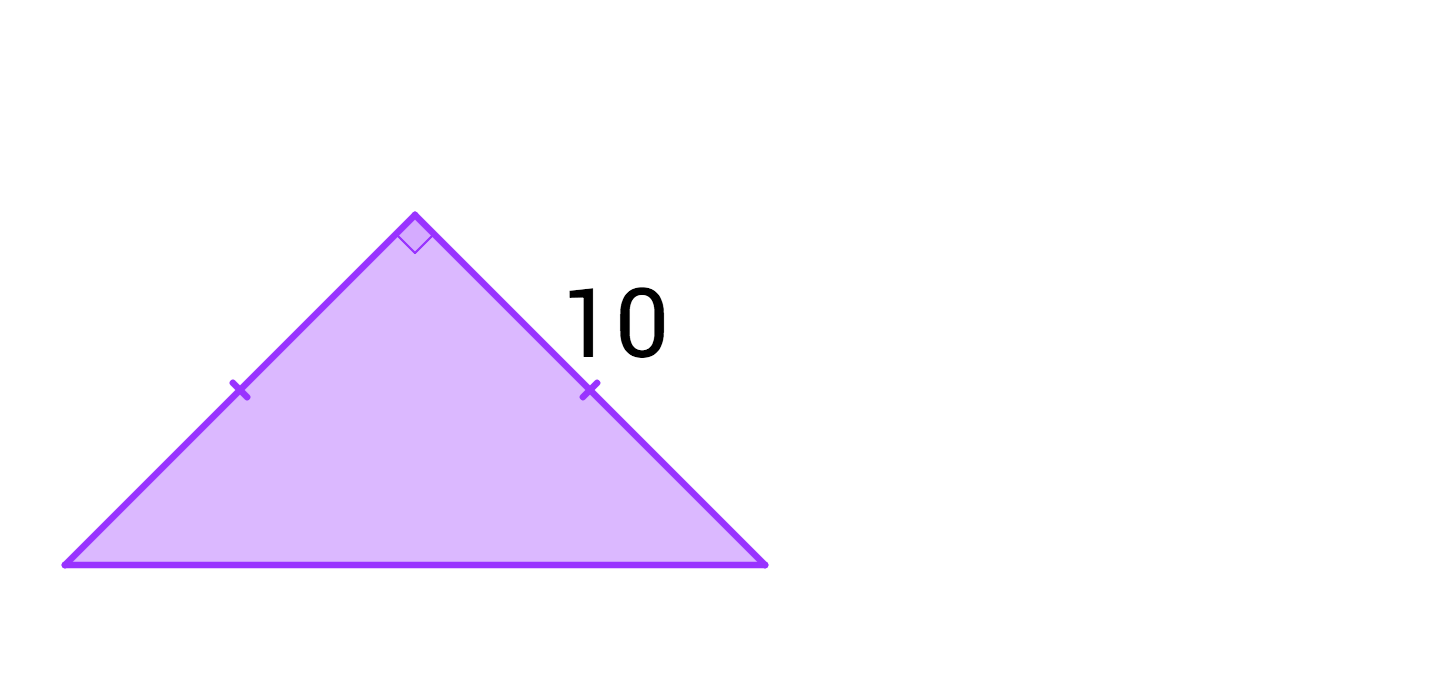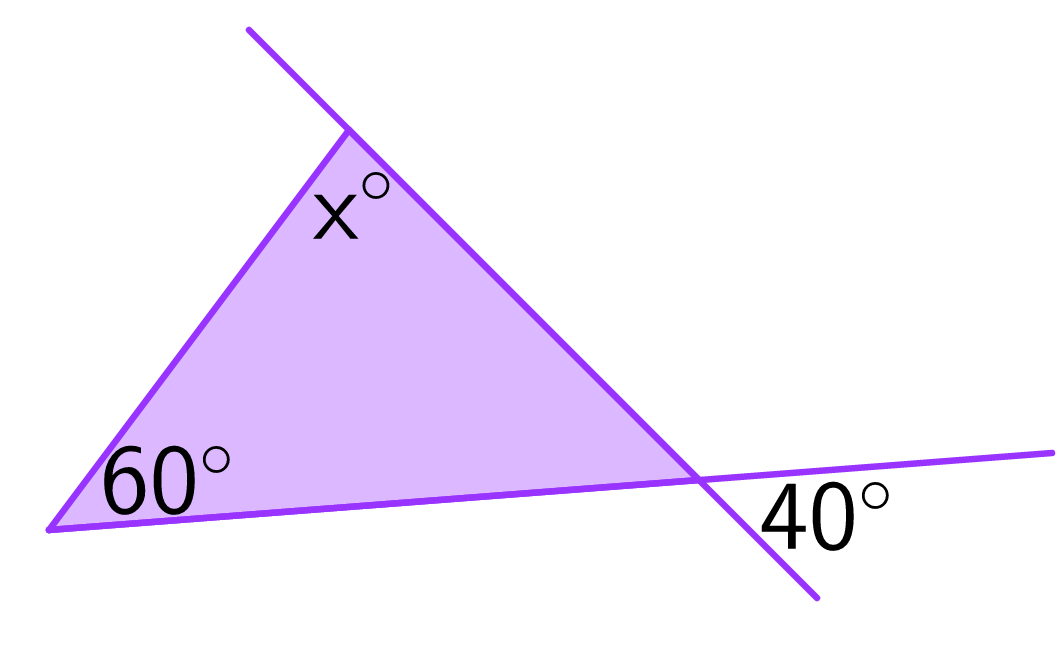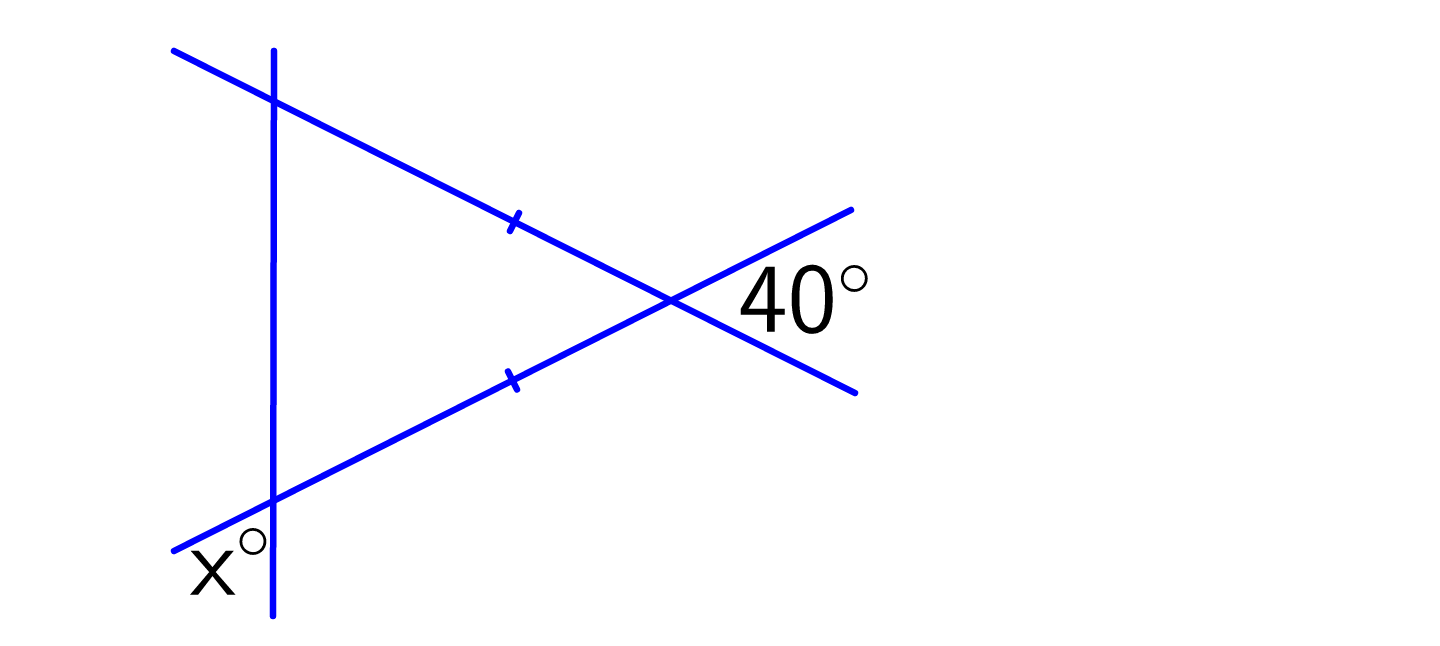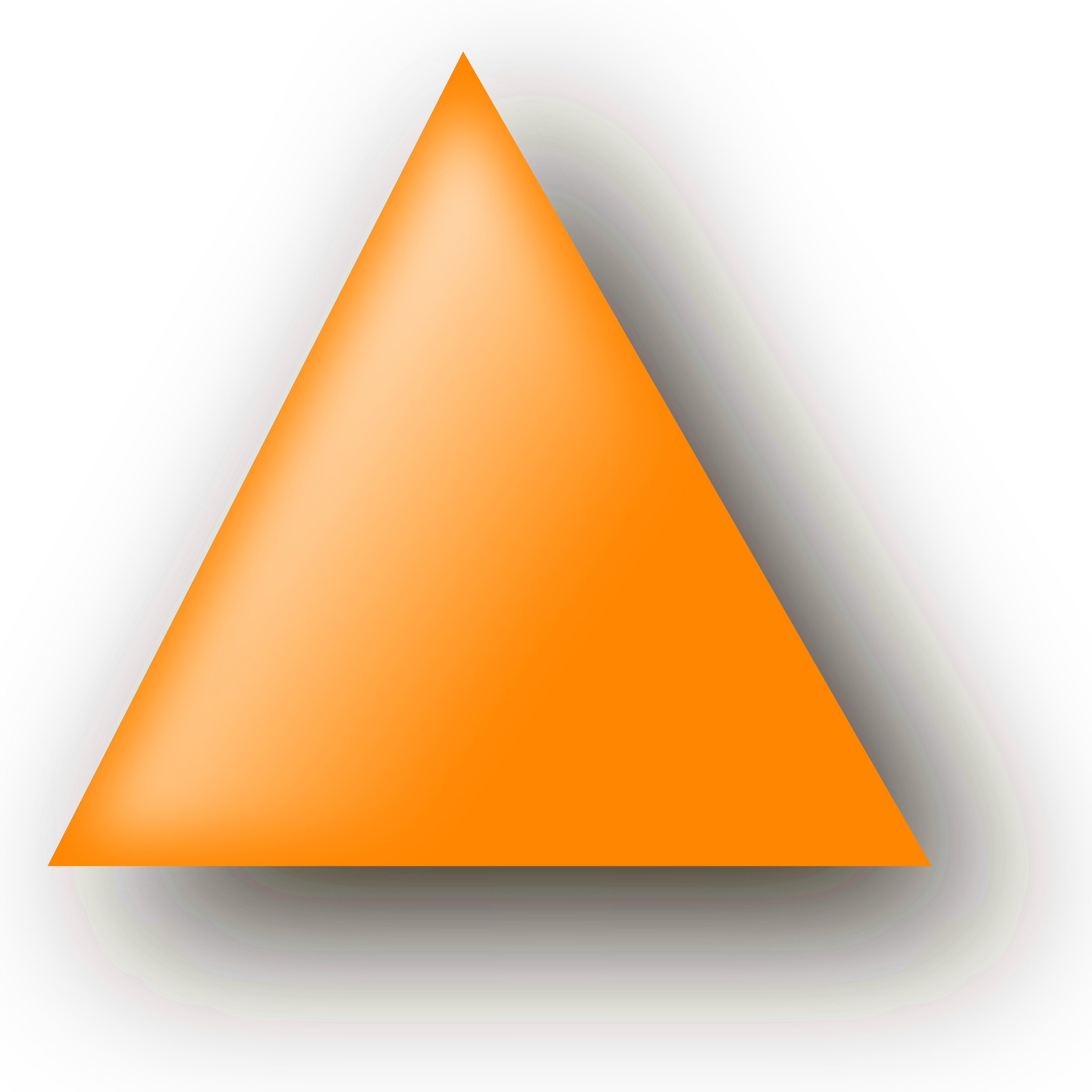Geometry

# Properties of Triangles Lightning QuizWhat is the area of the triangle?What is the value of $x?$

Vann's triangle has side lengths of $a, a + 1, \text{ and } a + 2.$ Which of these numbers could be the value of $a?$What is the value of $x?$If a triangle's height is doubled and its base length is halved, the area of the triangle will...

×